# 4th grade - math problems

Mathematical word problems allow you to practice your mathematics knowledge in everyday life tasks. Word problems train to understand, translate into the mathematical language (e.g., equations), solve it, and check the accuracy and solution discussion.
Choose a topic you want to calculate and improve in.

### From our database of math word problems and examples we offer:

• Two geometric progressions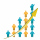Insert several numbers between numbers 6 and 384 so that they form with the given GP numbers and that the following applies: a) the sum of all numbers is 510 And for another GP to apply: b) the sum of entered numbers is -132 (These are two different geome
• Irregular pentagon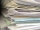A rectangle-shaped, 16 x 4 cm strip of paper is folded lengthwise so that the lower right corner is applied to the upper left corner. What area does the pentagon have?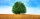The shadow of a 1 m high pole thrown on a horizontal plane is 0.8 m long. At the same time, the shadow of a tree thrown on a horizontal plane is 6.4 m. Determine the height of the tree.
• Price reduction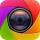The price of the camera is 654 USD on sale. They reduced its price to 411 USD. Calculate the percentage reduction in the price of the camera.
• Slope of trackCalculate the average slope (in permille and even in degrees) of the rail tracks between Prievidza (309 m AMSL) and Nitrianske Pravno (354 m AMSL), if the track is 11 km long.
• Non linear eqsSolve the system of non-linear equations: 3x2-3x-y=-2 -6x2-x-y=-7
• Markup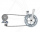A store pays \$370 for a bicycle.  The percent of markup is 19%.  What is the selling price of the bike?
• Sum of the seventeen numbersThe sum of the 17 different natural numbers is 154. Determine the sum of the two largest ones.
• The amphitheater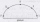The amphitheater has the shape of a semicircle, the spectators sit on the perimeter of the semicircle, the stage forms the diameter of the semicircle. Which of the spectators P, Q, R, S, T sees the stage at the greatest viewing angle?
• From plasticine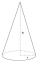Michael modeled from plasticine a 15 cm high pyramid with a rectangular base with the sides of the base a = 12 cm and b = 8 cm. From this pyramid, Janka modeled a rotating cone with a base diameter d = 10 cm. How tall was Janka's cone?
• Cans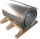How many m2 of metal sheet is needed to produce 20,000 cans in the shape of a cylinder with a base radius and a height of 5 cm.
• Three ints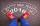The sum of three consecutive integers is 2016. What numbers are they?
• Diamond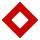The side length of the diamond is 35 cm, and the length of the diagonal is 56 cm. Calculate the height and length of the second diagonal.
• TyronTyron attended his online class 2 hours a day. He already attend 1 3/4 hours, how many more hours will Tyron attend his class?
• Bottles of juice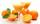How many 2-liter bottles of juice need to buy if you want to transfer juice to 50 pitchers rotary cone shape with a diameter of 24 cm and base side length of 1.5 dm.
• Simple equation 9Solve the following equation: -8y+5=-9y+9
• XY triangleDetermine the area of a triangle given by line 7x+8y-69=0 and coordinate axes x and y.